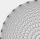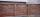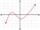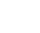# In the

In the arithmetic sequence a1 = 4.8, d = 0.4. How many consecutive members, starting with the first, need to be added so that the sum is greater than 170?

n =  20

### Step-by-step explanation:

Our quadratic equation calculator calculates it.Did you find an error or inaccuracy? Feel free to write us. Thank you!Tips to related online calculators

## Related math problems and questions:

• n-gonGabo draws n-gon, which angles are consecutive members of an arithmetic sequence. The smallest angle is 70° biggest 170°. How many sides have Gabo's n-gon?
• AS sequenceIn an arithmetic sequence is given the difference d = -3 and a71 = 455. a) Determine the value of a62 b) Determine the sum of 71 members.
• Finite arithmetic sequenceHow many numbers should be inserted between the numbers 1 and 25 so that all numbers create a finite arithmetic sequence and that the sum of all members of this group is 117?
• Phone numbersHow many 7-digit telephone numbers can be compiled from the digits 0,1,2,..,8,9 that no digit is repeated?
• The sumThe sum of the first 10 members of the arithmetic sequence is 120. What will be the sum if the difference is reduced by 2?
• SequenceBetween numbers 1 and 53 insert n members of the arithmetic sequence that its sum is 702.
• Consecutive numbersSum of ten consecutive numbers is 105. Determine these numbers (write first and last).
• Probability of intersectionThree students have a probability of 0.7,0.5 and 0.4 to graduated from university respectively. What is the probability that at least one of them will be graduated?
• SequenceIn the arithmetic sequence is a1=-1, d=4. Which member is equal to the number 203?
• Sum of membersWhat is the sum of the first two members of the aritmetic progression if d = -4.3 and a3 = 7.5?
• Sequence 3Write the first 5 members of an arithmetic sequence: a4=-35, a11=-105.
• Four integersFnd four consecutive integers so that the product of the first two is 70 times smaller than the product of the next two.
• Sorting ASCWhich group of decimals is in order from least to greatest? A) 1.04, 0.39, 0.8, 2.1, 0.09 B) 2.1, 1.04, 0.39, 0.8, 0.09 C) 0.09, 0.39, 0.8, 1.04, 2.1 D) 0.09, 0.39, 0.8, 2.1, 1.04
• Brick wallGarden 70 m long and 48 m wide should surround with wall 2.1 meters high and 30 cm thick. Wall will be built on the garden ground. How many will we need bricks if to 1 m³ is required approximately 300 bricks?
• EquationEequation f(x) = 0 has roots x1 = 64, x2 = 100, x3 = 25, x4 = 49. How many roots have equation f(x2) = 0 ?
• Chi squareA manufacturer of phone batteries claims that his batteries' lives are approximately normally distributed with a standard deviation equal to 0.9 years. If a random sample of 10 of these batteries has a standard deviation of 1.2 years. Do you think that th
• Stamps and albumsMichail has in three albums stored 170 stamps. In the first album, there are 14 more than in the second and in the second is by 1/5 less than in the third. How many stamps are in the first album?